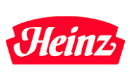# The Best Stocks With Dividend Growth From Last Week (May 21 – May 27, 2012)

57.12
TrefisHNZ
H.J. Heinz

Submitted by Dividend Yield as part of our contributors program.

Stocks With Biggest Dividend Hikes From Last Week by Dividend Yield – Stock, Capital, Investment. Here is a current sheet of companies that have announced a dividend increase within the recent week. In total, 21 stocks and funds raised dividends of which 14 have a dividend growth of more than 10 percent. The average dividend growth amounts to 19.29 percent. Exactly four stocks have a yield over five percent and seven are currently recommended to buy.

Here are the stocks with fast dividend growth:

Relevant Articles

H.J. Heinz Company (HNZ) has a market capitalization of \$17.11 billion. The company employs 34,800 people, generates revenues of \$11,649.08 million and has a net income of \$939.91 million. The firm’s earnings before interest, taxes, depreciation and amortization (EBITDA) amounts to \$1,746.87 million. Because of these figures, the EBITDA margin is 15.00 percent (operating margin 12.46 percent and the net profit margin finally 8.07 percent).

Financial Analysis: The company has USD 4.65 billion in long-term debt outstanding. Twelve trailing months earnings per share reached a value of \$2.85. Last fiscal year, the company paid \$1.92 in form of dividends to shareholders. The company announced to raise dividends by 7.3 percent.

Market Valuation: Here are the price ratios of the company: The P/E ratio is 18.77, P/S ratio 1.47 and P/B ratio is not calculable. Dividend Yield: 3.85 percent. The beta ratio is 0.53.

Hewlett-Packard Company (HPQ) has a market capitalization of \$44.15 billion. The company employs 349,600 people, generates revenues of \$127,245.00 million and has a net income of \$7,074.00 million. The firm’s earnings before interest, taxes, depreciation and amortization (EBITDA) amounts to \$14,661.00 million. Because of these figures, the EBITDA margin is 11.52 percent (operating margin 7.61 percent and the net profit margin finally 5.56 percent).

Financial Analysis: The total debt representing 23.65 percent of the company’s assets and the total debt in relation to the equity amounts to 79.31 percent. Due to the financial situation, a return on equity of 17.89 percent was realized. Twelve trailing months earnings per share reached a value of \$2.58. Last fiscal year, the company paid \$0.40 in form of dividends to shareholders. The company announced to raise dividends by 10.0 percent.

Market Valuation: Here are the price ratios of the company: The P/E ratio is 8.65, P/S ratio 0.35 and P/B ratio 1.15. Dividend Yield: 2.36 percent. The beta ratio is 1.08.

Bunge Limited (BG) has a market capitalization of \$8.91Billion. The company employs 32,000 people, generates revenues of \$58,743.00 million and has a net income of \$896.00 million. The firm’s earnings before interest, taxes, depreciation and amortization (EBITDA) amounts to \$1,685.00 million. Because of these figures, the EBITDA margin is 2.87 percent (operating margin 1.60 percent and the net profit margin finally 1.53 percent).

Financial Analysis: The total debt representing 17.53 percent of the company’s assets and the total debt in relation to the equity amounts to 34.86 percent. Due to the financial situation, a return on equity of 8.05 percent was realized. Twelve trailing months earnings per share reached a value of \$5.08. Last fiscal year, the company paid \$0.98 in form of dividends to shareholders. The company announced to raise dividends by 8.0 percent.

Market Valuation: Here are the price ratios of the company: The P/E ratio is 12.01, P/S ratio 0.15 and P/B ratio 0.81. Dividend Yield: 1.77 percent. The beta ratio is 1.19.

Ralph Lauren (RL) has a market capitalization of \$13.84 billion. The company employs 24,000 people, generates revenues of \$6,859.50 million and has a net income of \$681.00 million. The firm’s earnings before interest, taxes, depreciation and amortization (EBITDA) amounts to \$1,264.60 million. Because of these figures, the EBITDA margin is 18.44 percent (operating margin 15.15 percent and the net profit margin finally 9.93 percent).

Financial Analysis: The total debt representing 5.07 percent of the company’s assets and the total debt in relation to the equity amounts to 7.51 percent. Due to the financial situation, a return on equity of 19.58 percent was realized. Twelve trailing months earnings per share reached a value of \$7.13. Last fiscal year, the company paid \$0.80 in form of dividends to shareholders. The company announced to raise dividends by 100.0 percent.

Market Valuation: Here are the price ratios of the company: The P/E ratio is 21.00, P/S ratio 2.03 and P/B ratio 3.79. Dividend Yield: 1.07 percent. The beta ratio is 1.53.

Take a closer look at the full table of the stocks with recent dividend hikes. The average dividend growth amounts to 19.29 percent and the average dividend yield amounts to 2.54 percent. Stocks from the sheet are valuated with a P/E ratio of 15.86. The average P/S ratio is 1.75 and P/B 2.02.